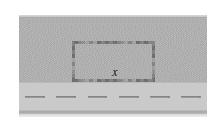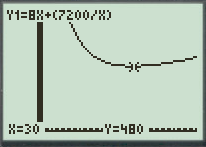# The model for the cost of fencing of the garden and the dimensions of garden that minimize the cost of fencing.### Precalculus: Mathematics for Calcu...

6th Edition
Stewart + 5 others
Publisher: Cengage Learning
ISBN: 9780840068071### Precalculus: Mathematics for Calcu...

6th Edition
Stewart + 5 others
Publisher: Cengage Learning
ISBN: 9780840068071

#### Solutions

Chapter 3.1, Problem 73E
To determine

## To find: The model for the cost of fencing of the garden and the dimensions of garden that minimize the cost of fencing.

Expert Solution

The model for the cost of fencing of the garden is equal to g(x)=8x+(7200x) and the dimensions of garden that minimize the cost of fencing are 30ft by 40ft .

### Explanation of Solution

Given information:

A property owner wants to fence a garden plot adjacent to a road, as shown in figure. The fencing next to the road must be sturdier and cost $5 per foot, but the other fencing costs just$3 per foot. The garden is to have an area of 1200ft2 .Calculation:

As given the area of the garden is 1200ft2 and the length of the fence along the road side is xft .

So, the breadth of the rectangular garden is equal to 1200xft .

The cost of the fence along the roadside is $5 per foot and the cost for fencing of other sides is equal to$3 per foot.

Evaluate the function for the total cost for fencing of garden.

g(x)=5x+3x+3×2(1200x)g(x)=8x+(7200x)

So, the function of the total cost of fencing the garden is given by g(x)=8x+(7200x) . To minimize the cost of fencing the rectangular region graph the function g(x)=8x+(7200x) .

To graph a function y=8x+(7200x) , follow the steps using graphing calculator.

First press “ON” button on graphical calculator, press Y= key and enter right hand side of the equation y=8x+(7200x) after the symbol Y1 . Enter the keystrokes 8X+(7200÷X) .

The display will show the equation,

Y1=8X+(7200/X)

Now, press the GRAPH key and TRACE key to produce the graph of given function in standard window as shown in Figure (1).Figure (1)

As observed from the graph, the cost function has minimum value equal to \$480 at x=30 .

So, the length of the region is equal to 30ft and breadth of the region is equal to 120030=40ft .

Therefore, the model for the cost of fencing of the garden is equal to g(x)=8x+(7200x) and the dimensions of garden that minimize the cost of fencing are 30ft by 40ft .

### Have a homework question?

Subscribe to bartleby learn! Ask subject matter experts 30 homework questions each month. Plus, you’ll have access to millions of step-by-step textbook answers!